数据挖掘项目：银行信用评分卡建模分析（上篇）

3 篇文章 1 订阅

kaggle上的Give Me Some Credit一个8年前的老项目，网上的分析说明有很多，但本人通过阅读后，也发现了很多的问题。比如正常随着月薪越高，违约率会下降。但对于过低的月薪，违约率却为0等。

• 上篇
1. 理解数据
2. 探索性数据分析
3. 数据预处理
• 下篇
1. 特征工程
2. 构建模型
3. 模型评估
4. 评分卡建立

1.理解数据

导入数据

# 需要导入的包
import pandas as pd
import numpy as np
import matplotlib.pyplot as plt
import seaborn as sns
import datetime
import scipy
%matplotlib inline
sns.set(style="ticks")
pd.set_option("display.max_columns",None)#展示所有列数据
pd.set_option("display.max_rows",None)#展示所有行数据
# 画图显示中文
plt.rcParams['font.sans-serif']=['SimHei']
plt.rcParams['axes.unicode_minus'] = False
from sklearn.ensemble import RandomForestRegressor as rfr
from imblearn.over_sampling import SMOTE
from sklearn.linear_model import LogisticRegression as LR
import scikitplot as skplt

查看数据基本信息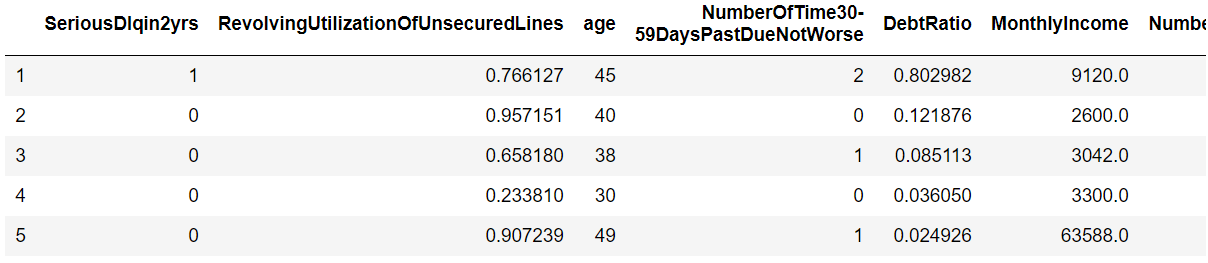：对测试数据也做同样的操作，这里只举例训练数据。

train.shape
(150000, 11)
test.shape
(101503, 11)

数据特征含义

• SeriousDlqin2yrs -----出现 90 天或更长时间的逾期行为（即定义好坏客户）
• RevolvingUtilizationOfUnsecuredLines ----- 贷款以及信用卡可用额度与总额度比例
• age ----- 借款人借款年龄
• NumberOfTime30-59DaysPastDueNotWorse----- 过去两年内出现35-59天逾期但是没有发展得更坏的次数
• DebtRatio----- 每月偿还债务，赡养费，生活费用除以月总收入
• MonthlyIncome -----月收入
• NumberOfOpenCreditLinesAndLoans -----开放式贷款和信贷数量
• NumberOfTimes90DaysLate -----过去两年内出现90天逾期或更坏的次数
• NumberRealEstateLoansOrLines -----抵押贷款和房地产贷款数量，包括房屋净值信贷额度
• NumberOfTime60-89DaysPastDueNotWorse -----过去两年内出现60-89天逾期但是没有发展得更坏的次数
• NumberOfDependents -----家庭中不包括自身的家属人数（配偶，子女等）

查看描述统计信息

train.describe([0.01,0.03,0.05,0.07,0.1,0.25,.5,.75,.9,.99]).T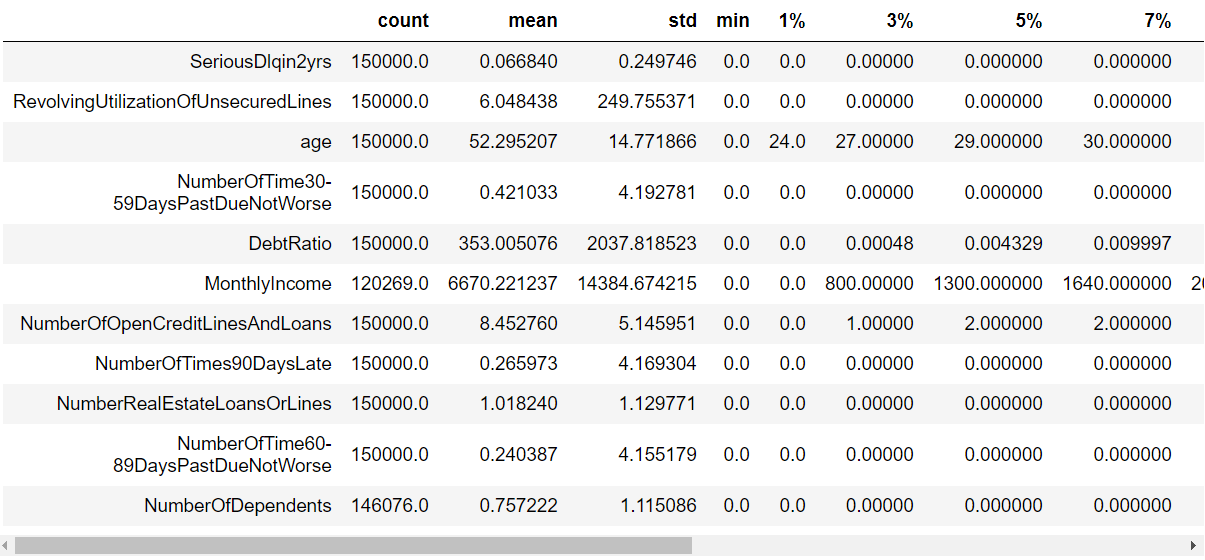• 有多个特征存在异常值。比如贷款额度与总额度比例存在大于1甚至几千的情况最大值为50708。年龄最小值为0，最大值为109的情况等等。这些异常值在数据探索性分析中将逐个进行说明。
• 多个特征存在有偏分布。比如贷款额度与总额度，月薪，负债率等特征。
train.info()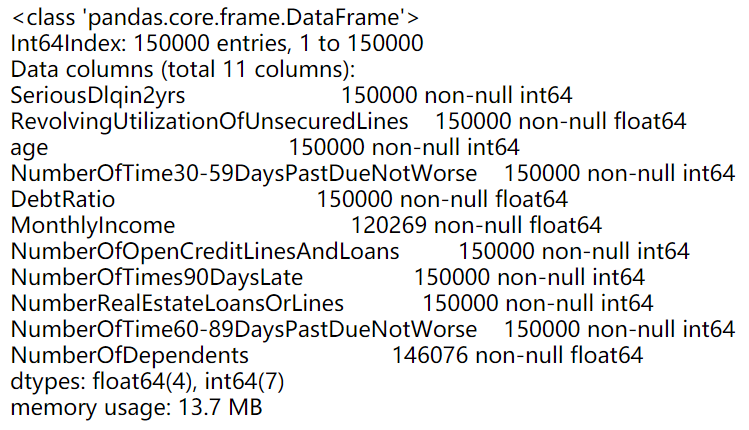• 数据类型都为int或float，说明都是数值型的数据。
• 月薪和家属人数存在空缺值。

2.探索性数据分析

目标特征分析：好坏客户特征

figure,ax = plt.subplots(figsize=(12,4))
train['SeriousDlqin2yrs'].value_counts().plot.pie(autopct='%1.1f%%')
plt.show()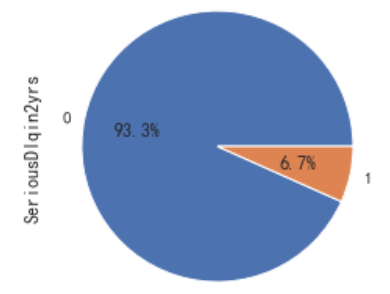• 正样本（好客户）占比93.3%，负样本（坏客户）占比6.7%。说明客户一般为好客户。我们需要捕捉的是坏客户，但由于比例太过悬殊，此时样本不平衡，需要后续进行处理。

贷款额度与总额度比例

fig= plt.figure(figsize=(14,4))
sns.distplot(train['RevolvingUtilizationOfUnsecuredLines'],kde=True)
sns.boxplot(y=train['RevolvingUtilizationOfUnsecuredLines'])
plt.show()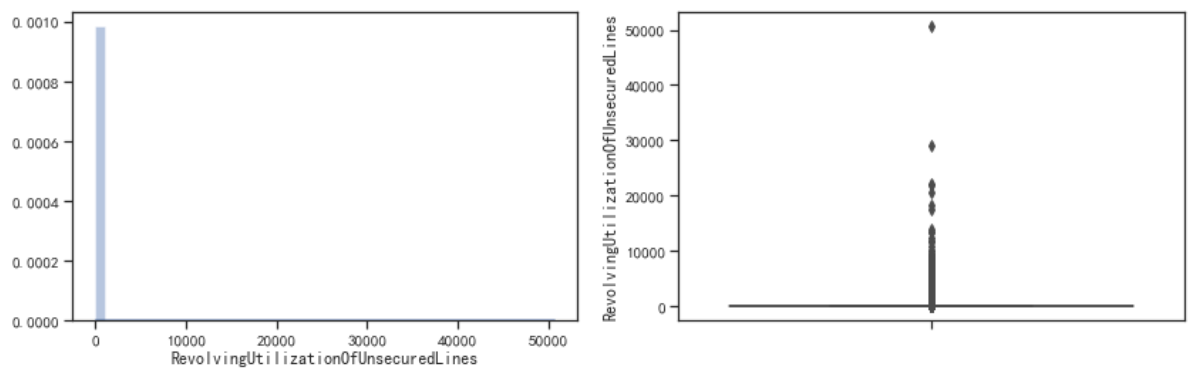• 样本分布极度不平衡。大部分的值都在“0左右”，存在大量的异常数据。需要进行进一步的分析。
• 而这些异常数据可能是由于没有除以总额度造成的，或是别的一些情况。这就需要借助具体的业务进行分析。

cut_num=[0,0.3,0.5,0.7,1,10,100,1000,10000]
get_compare_plot(train,feature_plot="RevolvingUtilizationOfUnsecuredLines",cut_num=cut_num,is_qcut=False)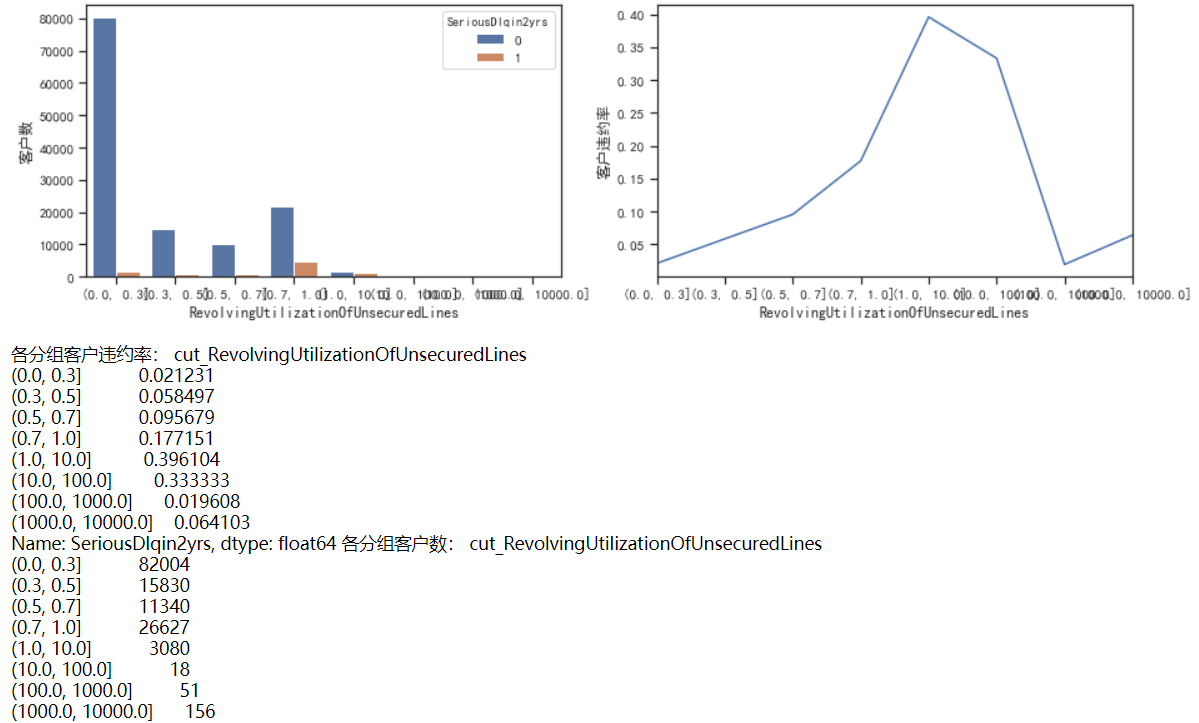• 这里可以看到，当Revol(贷款额度与总额度比例) 小于1时，随着该比例的增加，客户违约率也在增加，符合业务逻辑。正常来说，应该是随着比例的增加，客户违约率也会增加，但是在大于1后，该规律发生了改变，因此对其进行进一步分析。
cut_num=[0,1,10,30,50,70,100,1000,10000,100000]
get_compare_plot(train,feature_plot="RevolvingUtilizationOfUnsecuredLines",cut_num=cut_num,is_qcut=False)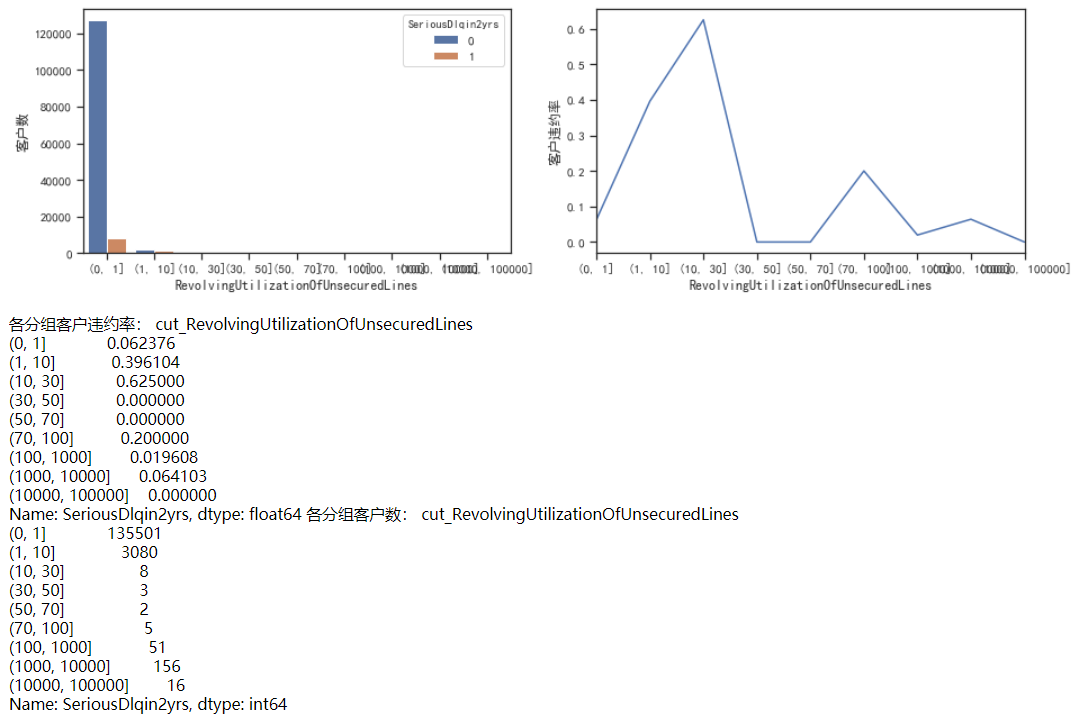• 这里主要分析Revol大于10是什么情况。通过图像和数据可以发现，当比例大于10后，客户违约率在10~30达到高峰，然后下降，再往后的比例都差不多，上下浮动。
• 出现这个情况，此时要做的就是找到阈值，能分割正常值和异常值的阈值。我们可以认定10~30是一个分界点，因为它的违约率非常高，但是要注意，10-30这个区间内，客户数只有8人，随机性太大，因此不做考虑。发现1-10这个区间内的样本数足够，并且违约率也在上升，因此进一步分析。
cut_num=[]
for i in np.arange(-1,11,1):
cut_num.append(i)
get_compare_plot(train,feature_plot="RevolvingUtilizationOfUnsecuredLines",cut_num=cut_num,is_qcut=False)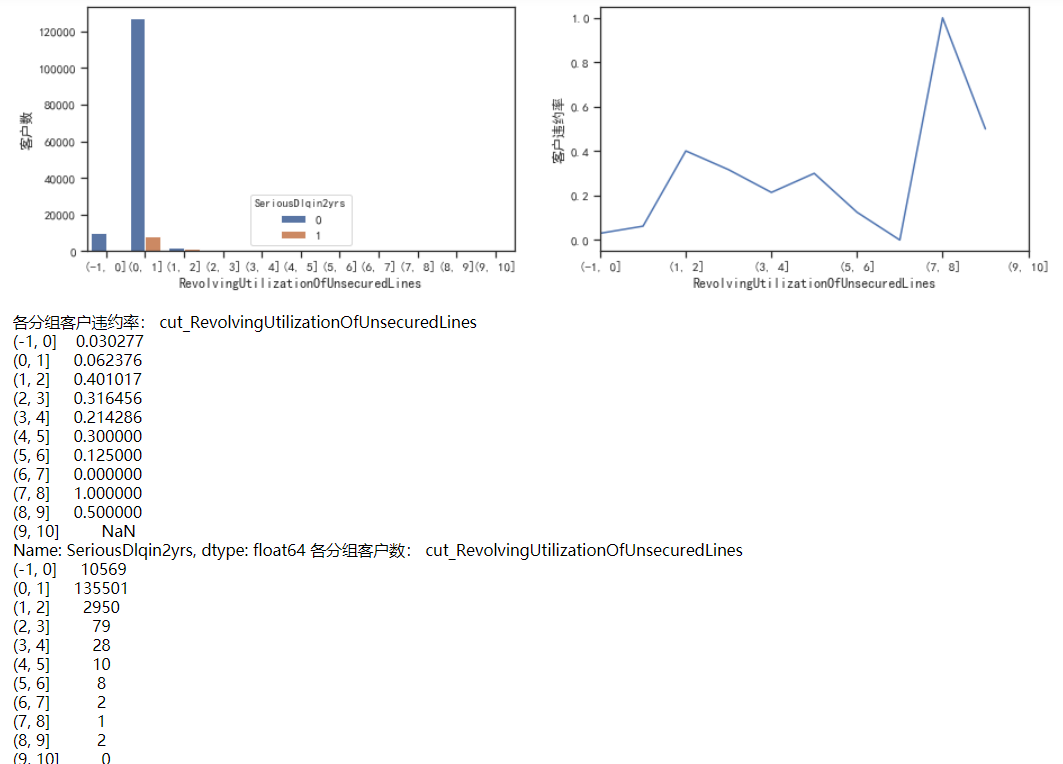• 对1-10分析后，可以看到，在数据量足够的情况下（大于30），即1-4，违约率是在逐渐下降的，这不符合正常的规律性，应该是Revol越大，违约率越大。
• 我们发现在1-2时，违约率有了特别大的提升，从0.06到0.4。因此，可以认为阈值在这个区间内。
cut_num=[]
for i in np.arange(0.6,3.2,0.2):
cut_num.append(i)
get_compare_plot(train,feature_plot="RevolvingUtilizationOfUnsecuredLines",cut_num=cut_num,is_qcut=False)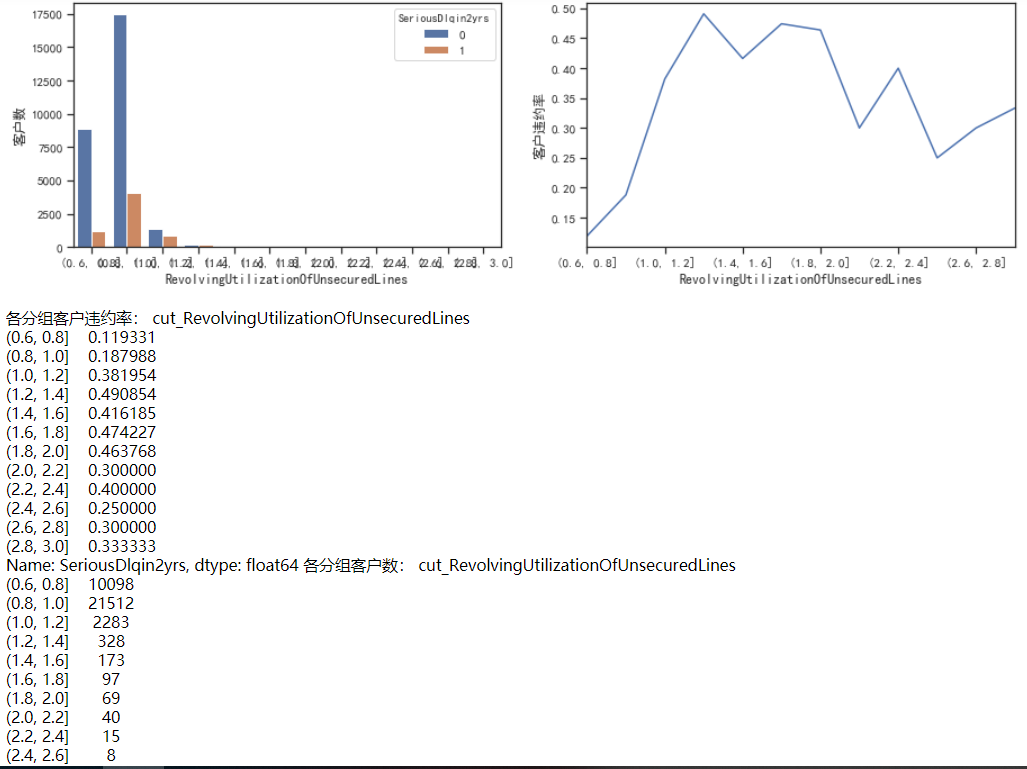• 之间对0-1之间做过分析，因此从0.6开始。
• 通过和之前的分析对比来看，Revol在0~2内，违约率增加。超过2之后，客户数量迅速下降和违约率也有降低的趋势。因此，可以认为Revol=2，是我们要找的阈值。大于2的为异常值，小于2的为正常值。
print("贷款以及信用卡可用额度与总额度比例大于2的比重为：",100*(train['RevolvingUtilizationOfUnsecuredLines']>2).sum()/len(train),"%")

• 大于2的异常值，我们可以删除。如下其占比非常低0.247%。也可以融到0~2的数据内。想到的方法有两个：一是对大于2的值统一进行缩放；二是分组，直接把大于2的值分组到0-1内。这里采用第二种分箱的方法，因为分箱的一个特点就是可以用来处理异常值。
cut_num=[-1,0,1,2,100000]
get_compare_plot(train,feature_plot="RevolvingUtilizationOfUnsecuredLines",cut_num=cut_num,is_qcut=False)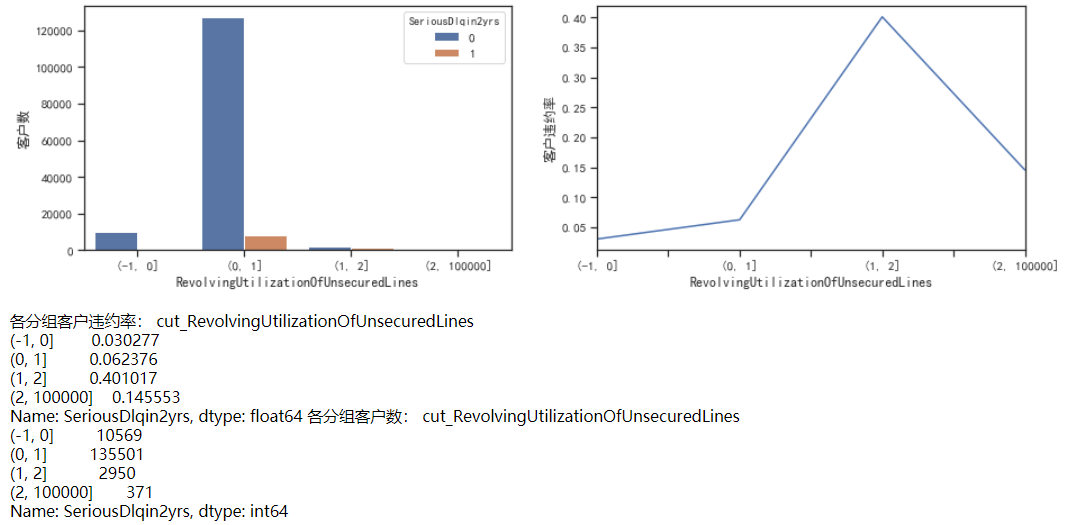• 大于2的样本有371个。将该组与0-1进行合并。原因有两点：一是0-1数据量大，抗随机性强。不会因为371个样本产生太大的波动。二是因为这371个样本，如果正常计算，归属于0-1的概率最大，因为0-1样本占比最大。因此与0-1组进行合并

年龄

fig= plt.figure(figsize=(14,4))
sns.distplot(train['age'],kde=True)
sns.boxplot(y=train['age'])
plt.show()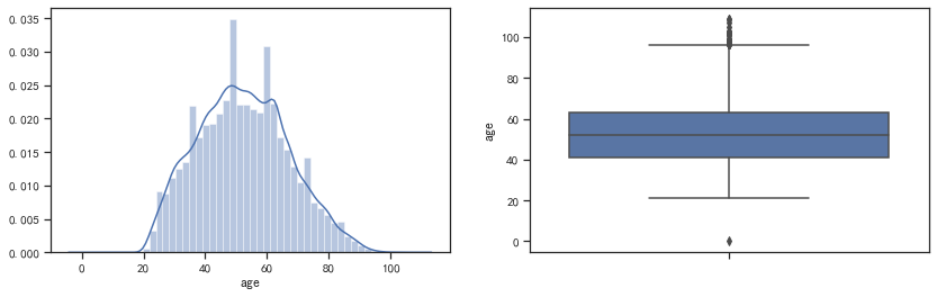• 这里年龄的分布比较接近正态分布，因此，先使用四分位差的方法来找寻异常值。（上下四分位数±1.5~3倍的四分位差）
age_mean=train['age'].mean()
age_std=train['age'].std()
age_lowlimit=age_mean-3*age_std
age_uplimit=age_mean+3*age_std
print('异常值下限：',age_lowlimit,'异常值上限：',age_uplimit)

• 发现年龄的下限为8岁，上限为97岁。应用四分位差的方法来找寻异常值的一个前提条件是，数据最好服从正态分布，否则会有一些偏差。这里计算一下该数据的偏度和峰度。
print('峰度：',train['age'].skew(),'偏度：',train['age'].kurt())

• 发现峰度较小，偏度为负数，SK=-0.494，SK接近-0.5，即中度左偏分布。因此，用这个四分位差去判断不是很准确。接下来对图形进行分析，进一步判断异常值。
cut_num=[20,30,40,50,60,70,80,90,100,110]
get_compare_plot(train,feature_plot="age",cut_num=cut_num,is_qcut=False)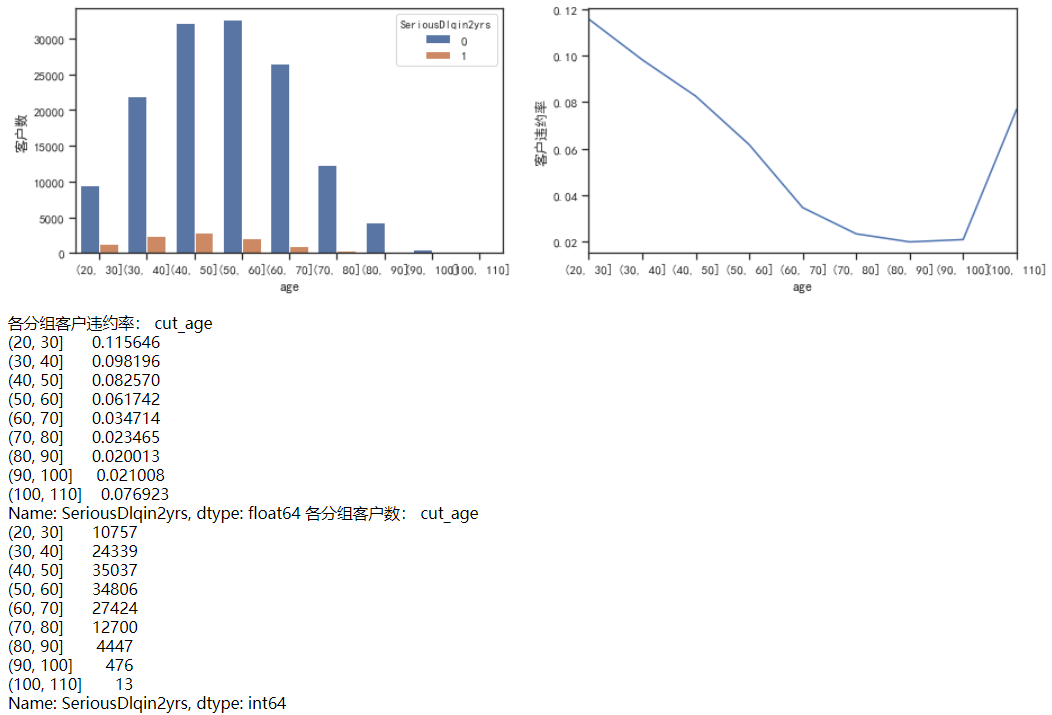• 把年龄从20-110每10岁分一组。发现随着年龄的增加，客户违约率在逐渐降低。
1.（年龄越大，越不容易违约）但在100-110这个阶段，违约率上升，不符合规律。
2.年龄过大（100岁以上贷款），这种情况也比较少见。
3.之前的97岁，由于数据非正态分布，会出现偏差。如果以100为分界点，数比较整，也好处理。
因此，基于以上3个原因，选择100为异常值的阈值，大于100的删除

过去两年内出现35-59天|60-89天|90天以上|逾期但是没有发展得更坏的次数

fig,[[ax1,ax2],[ax3,ax4],[ax5,ax6]] = plt.subplots(3,2,figsize=(20,15))
sns.distplot(train['NumberOfTime30-59DaysPastDueNotWorse'],ax=ax1)
sns.boxplot(y=train['NumberOfTime30-59DaysPastDueNotWorse'],ax=ax2)
sns.distplot(train['NumberOfTime60-89DaysPastDueNotWorse'],ax=ax3)
sns.boxplot(y=train['NumberOfTime60-89DaysPastDueNotWorse'],ax=ax4)
sns.distplot(train['NumberOfTimes90DaysLate'],ax=ax5)
sns.boxplot(y=train['NumberOfTimes90DaysLate'],ax=ax6)
plt.show()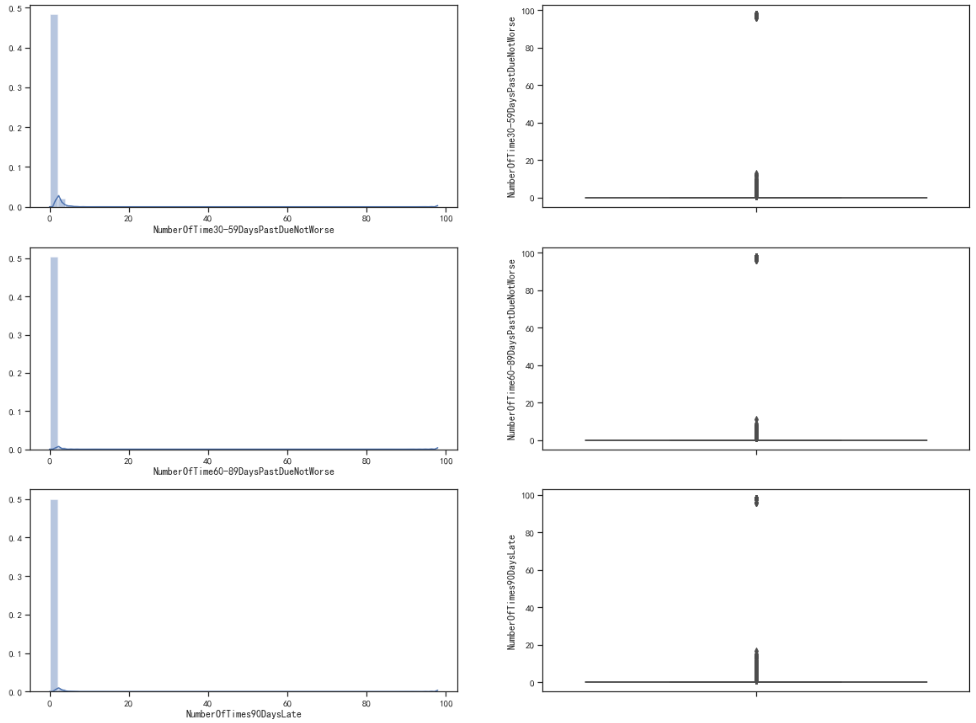• 通过分析可以发现，绝大多数值都在“0附近”，但也有次数超过80的异常值。

• 30~59天24次。
• 60~89天最多的违规次数为12次。
• 90天以上最多的违规次数为8次。
print('两年内30~59天违规次数超过24次的样本数为：',(train['NumberOfTime30-59DaysPastDueNotWorse']>24).sum())
print('两年内60-89天违规次数超过12次的样本数为：',(train['NumberOfTime60-89DaysPastDueNotWorse']>12).sum())
print('两年内90天以上违规次数超过8次的样本数为：',(train['NumberOfTimes90DaysLate']>8).sum())

• 以上是训练集数据中出现的超过限定范围的样本数。将以上数据删除处理。
train["qcut_30-59"], updown = pd.qcut(train["NumberOfTime30-59DaysPastDueNotWorse"], retbins=True, q=20,duplicates='drop')
train["qcut_60-89"], updown = pd.qcut(train["NumberOfTime60-89DaysPastDueNotWorse"], retbins=True, q=20,duplicates='drop')
train["qcut_90"], updown = pd.qcut(train["NumberOfTimes90DaysLate"], retbins=True, q=20,duplicates='drop')

fig = plt.figure(figsize=(18,4))
(train.groupby('qcut_30-59')['SeriousDlqin2yrs'].sum()/train.groupby('qcut_30-59')['SeriousDlqin2yrs'].count()).plot()
(train.groupby('qcut_60-89')['SeriousDlqin2yrs'].sum()/train.groupby('qcut_60-89')['SeriousDlqin2yrs'].count()).plot()
(train.groupby('qcut_90')['SeriousDlqin2yrs'].sum()/train.groupby('qcut_90')['SeriousDlqin2yrs'].count()).plot()
plt.show()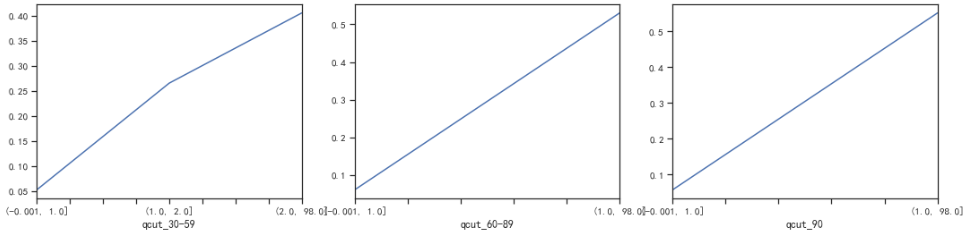• 逾期的次数越多，客户违约率就越高，符合正常逻辑。

负债率

fig= plt.figure(figsize=(14,4))
sns.distplot(train['DebtRatio'],kde=True)
sns.boxplot(y=train['DebtRatio'])
plt.show()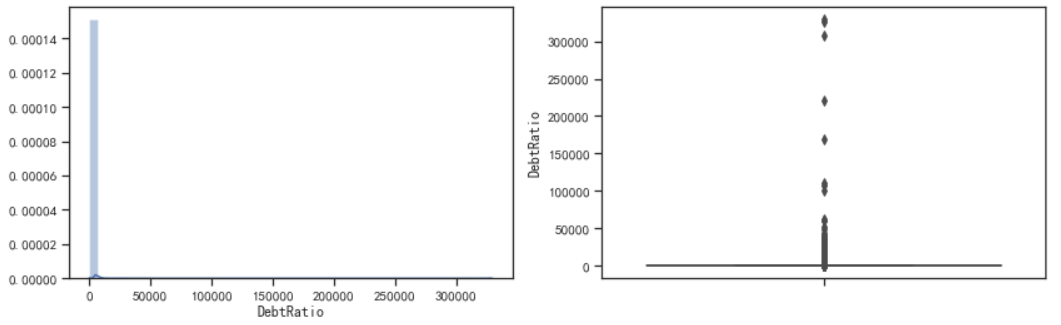• 负债率主要集中在“0附近”，正常来说，负债率应该是小于1的。但却有很多的值大于1，甚至上万，即存在异常值。
cut_num=[-1,0,0.3,0.5,0.7,1,10,100,1000,10000]
get_compare_plot(train,feature_plot="DebtRatio",cut_num=cut_num,is_qcut=False)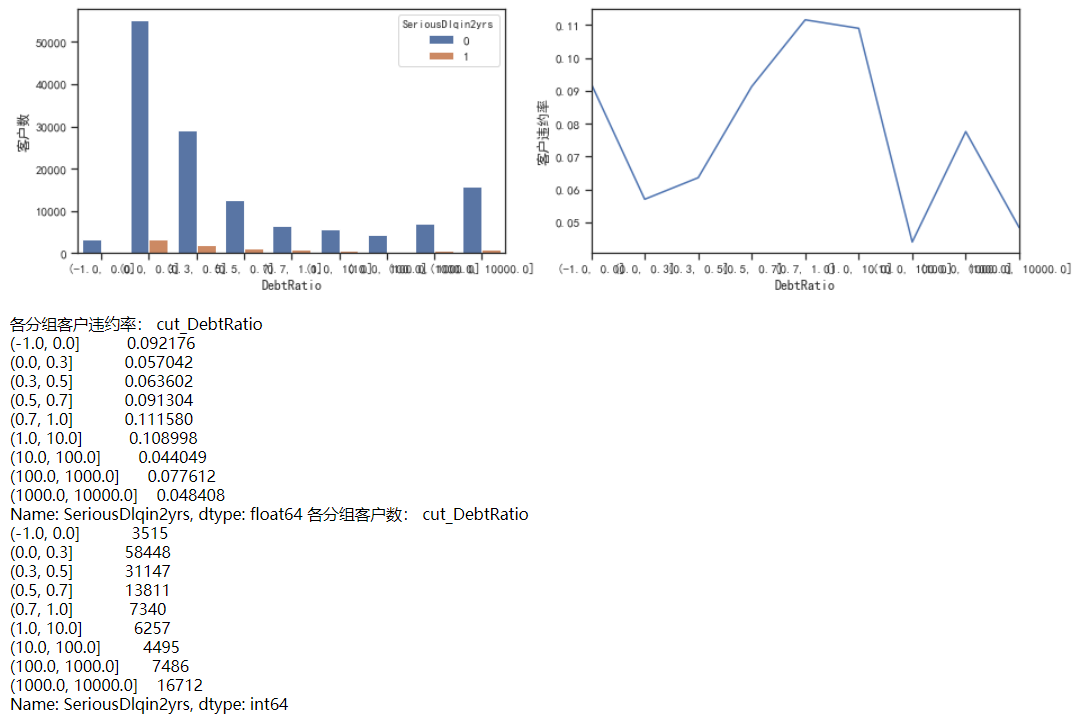• 负债率在0-1之间，随着负债率的增加，违约率也在增加。
• 当负债率为0时，有着较高的违约率，这点需要从业务上去理解。可能是因为若无负债（每月偿还债务，赡养费，生活费用除以月总收入），比较不靠谱。
• 当负债率大于1时，随着负债率的增加，违约率无显著的变化规律。这里对大于1继续进行分析。
cut_num=[10,30,50,70,100]
get_compare_plot(train,feature_plot="DebtRatio",cut_num=cut_num,is_qcut=False)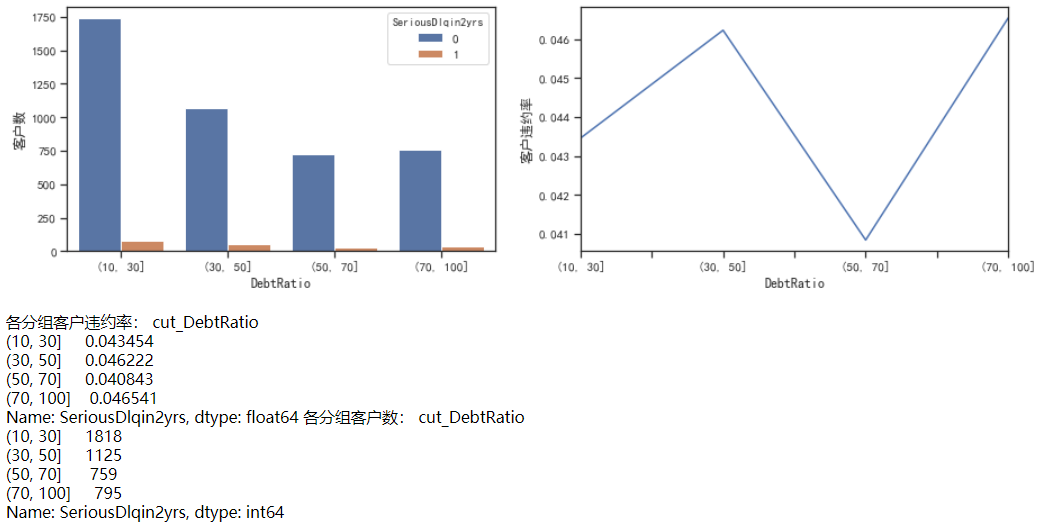• 负债率在10-100之间时，没有逻辑规律，虽然从图像上看数据变化较大，但违约率都在0.04左右，很低
cut_num=[]
for i in np.arange(0,11,1):
cut_num.append(i)
get_compare_plot(train,feature_plot="DebtRatio",cut_num=cut_num,is_qcut=False)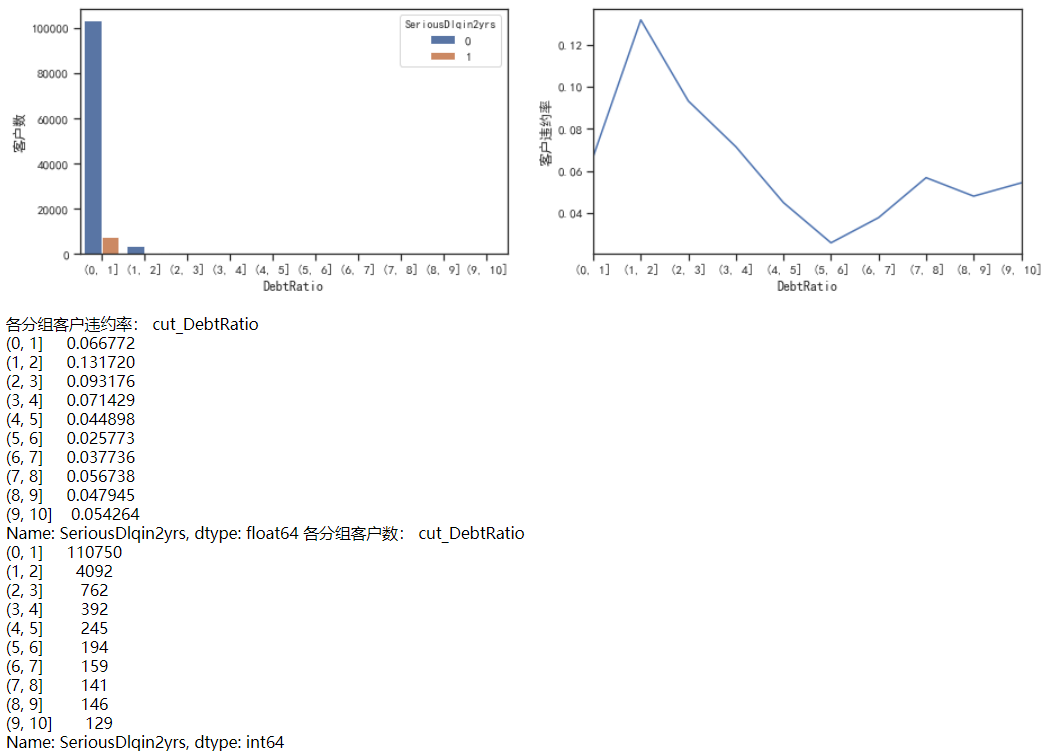• 负债率在0-2时，随着负债率的增加，违约率也在增加。但大于2之后，负债率下降，然后在0.03-0.05上下波动。
• 可认定2为阈值，大于2的为异常值。但这个异常值的处理却有些问题，跟月薪的关系很大。

月薪

a=(len(train)-len(train_nM))/len(train)*100
print('家属人数缺失值比例为%.2f%%'%(a))

• 之前说过，月薪存在缺失值，这里可看到缺失值的比例为19.82%。数值很大，采用随机森林进行填充。
fig= plt.figure(figsize=(14,4))
sns.distplot(train_nM['MonthlyIncome'],kde=True)
sns.boxplot(y=train_nM['MonthlyIncome'])
plt.show()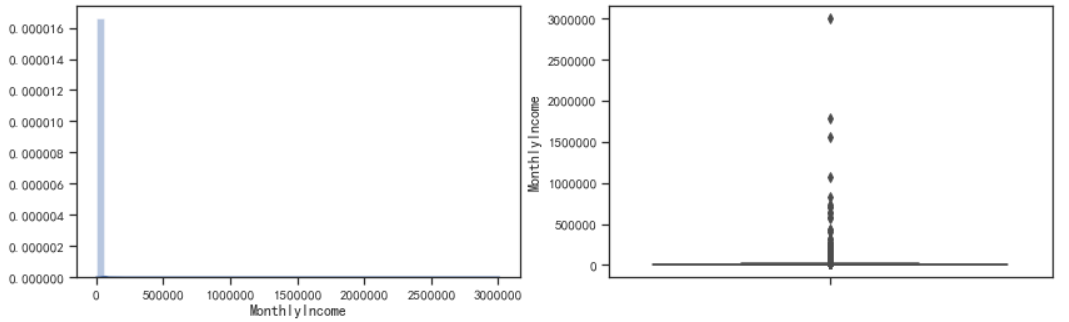• 月薪的分布也是极度右偏的。有数额特别大的异常值。
cut_num=[0,1000,5000,10000,15000,20000,100000]
get_compare_plot(train,feature_plot="MonthlyIncome",cut_num=cut_num,is_qcut=False)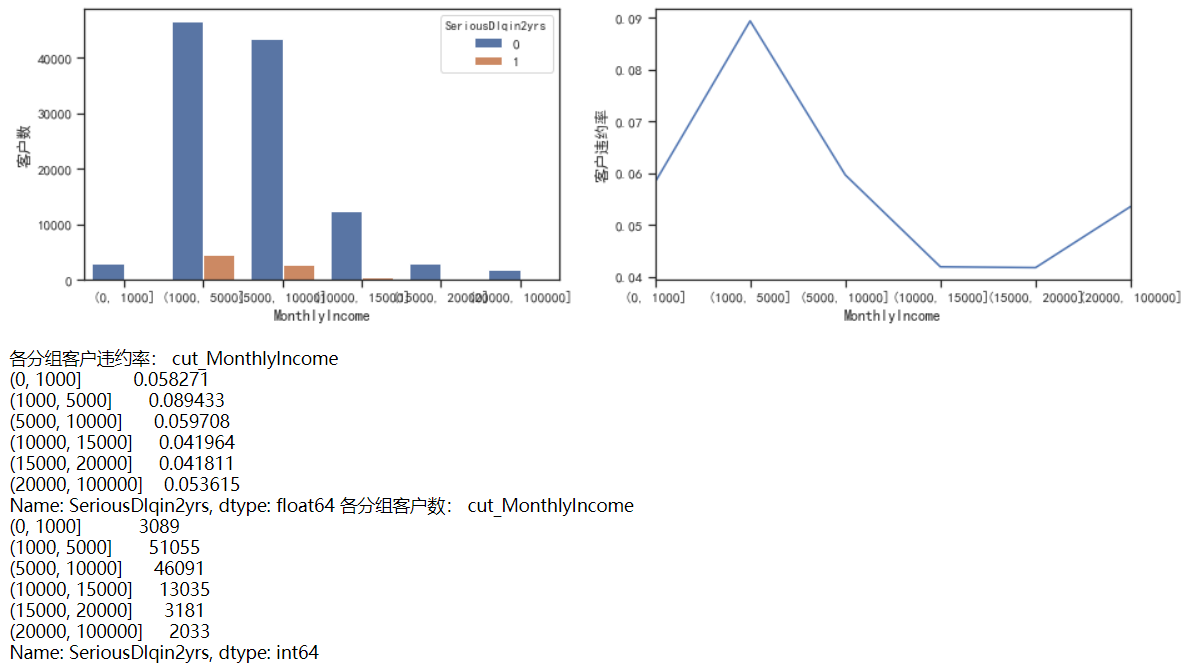• 随着月薪的增加，客户违约率逐渐下降。当月薪超过20000后，违约率又有上升趋势，说明月薪太大，客户收入不稳定。
• 注意到0-1000这个月薪范围内跟正常的规律不一样。进行进一步的判断。
train_nM_2=train_nM.loc[(train_nM['MonthlyIncome']>1)&(train_nM['MonthlyIncome']<=3000),:]
sns.scatterplot(x='MonthlyIncome',y='DebtRatio',data=train_nM_2,hue='SeriousDlqin2yrs')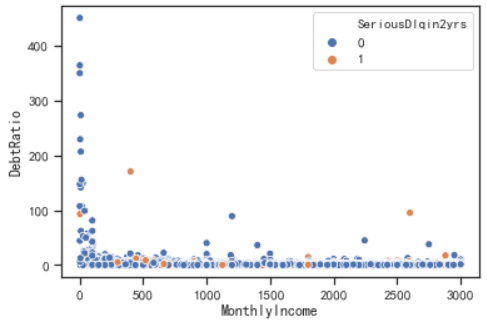• 绘制月薪和负债率的散点图，发现500-3000月薪的负债率都很低，有比较稳定的规律性，但是0-500之间却很高。因此对0-500进行进一步分析。
train_nM_2=train_nM.loc[(train_nM['MonthlyIncome']>1)&(train_nM['MonthlyIncome']<700),:]
sns.scatterplot(x='MonthlyIncome',y='DebtRatio',data=train_nM_2,hue='SeriousDlqin2yrs')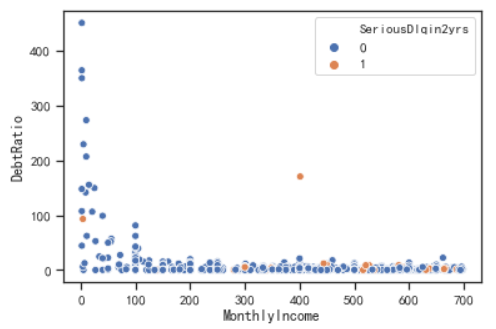• 月薪在0-100之间，负债率特别高，但是违约率却很低说明月薪在这里被算小了，可能是客户在输入金额时把自己的年薪输入进去，由于输入金额时，差的都是1000倍。因此，将0-100之间的金额乘1000再除以12，以求出正常的月薪，同时负债率也要跟着一起变化0.012倍。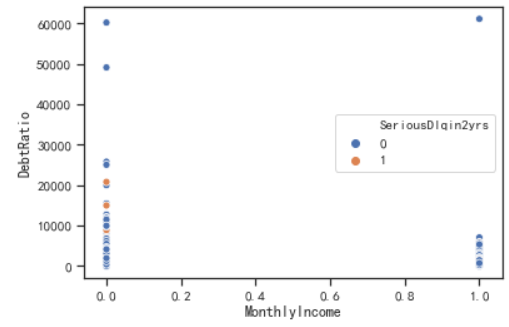开放式贷款和信贷数量

fig= plt.figure(figsize=(14,4))
sns.distplot(train['NumberOfOpenCreditLinesAndLoans'],kde=True)
sns.boxplot(y=train['NumberOfOpenCreditLinesAndLoans'])
plt.show()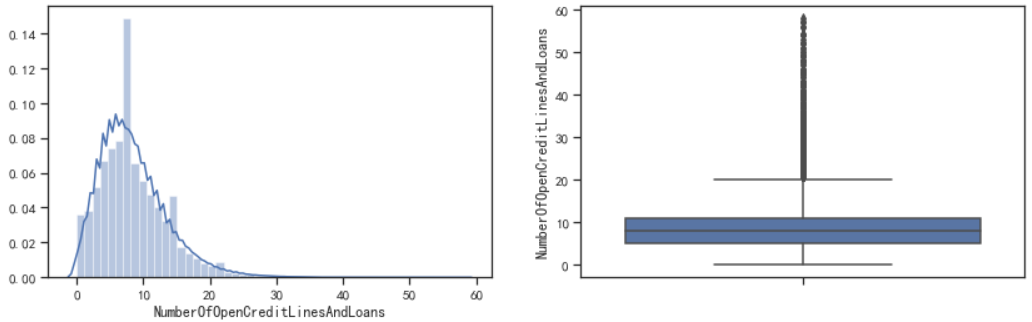• 通过直方图可以看到，数据是右偏分布，可能存在异常值。
• 通过箱线图可以看到，存在一些离散值。但由于这些离散值较多且连续，因此需要进一步判断是不是异常值。
cut_num=[-1,0,1,3,5,7,10,20,30,40]
get_compare_plot(train,feature_plot="NumberOfOpenCreditLinesAndLoans",cut_num=cut_num,is_qcut=False)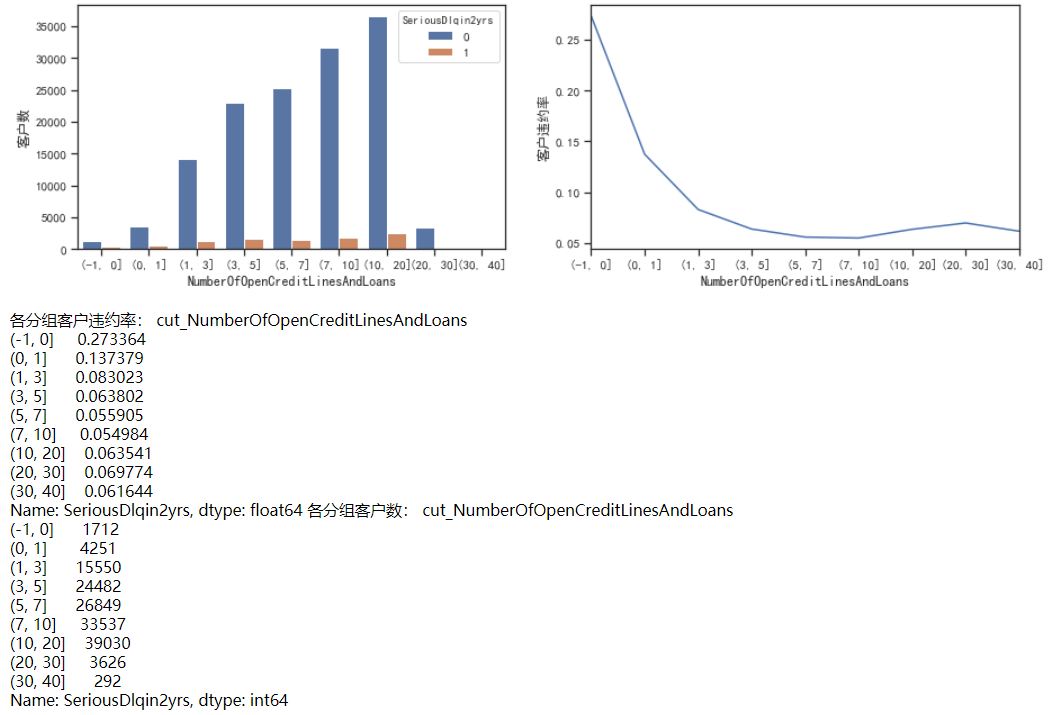• 随着信贷数量增加，客户违约率在不断降低，最后基本保持在0.06左右。即信贷数量越多，说明该客户越值得信赖。
• 这里可以认定大于30的为异常值，因为30-40的样本量突然下降。
• 但也可以通过箱线图看出，30-40的值为连续的，没有断点。这里先不按照异常值进行处理

抵押贷款和房地产贷款数量

fig= plt.figure(figsize=(14,4))
sns.distplot(train['NumberRealEstateLoansOrLines'],kde=True)
sns.boxplot(y=train['NumberRealEstateLoansOrLines'])
plt.show()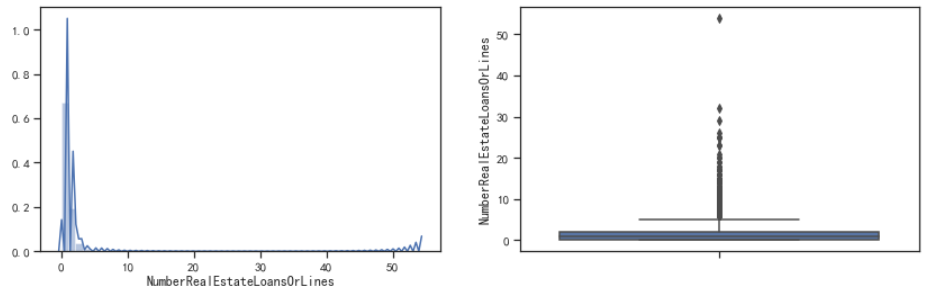• 通过直方图能看到数据是极度右偏的分布，另外箱线图上也能明显地看到离群点。
cut_num=[-1,0,1,3,5,7,10,15,20,25,50]
get_compare_plot(train,feature_plot="NumberRealEstateLoansOrLines",cut_num=cut_num,is_qcut=False)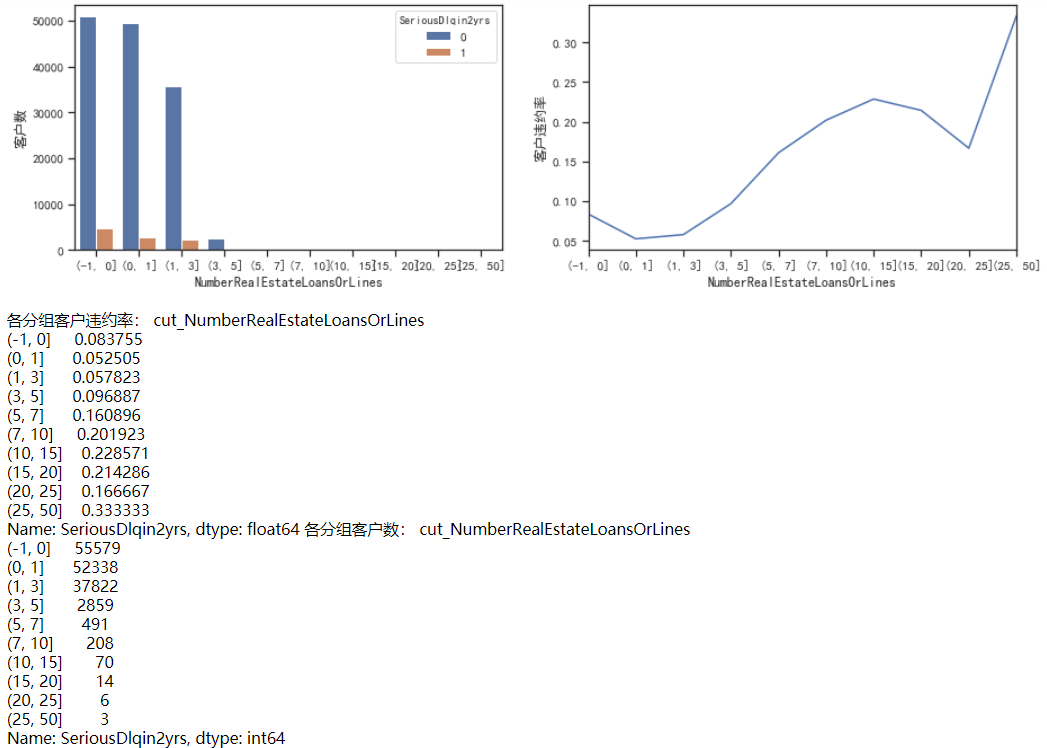• 当抵押贷款和房地产贷款数量为0时，客户有一定的违约率，跟之前的负债率一样。
• 当抵押贷款和房地产贷款数量大于0，小于20时，随着数量的增加，客户违约率也在不断增加。
• 由于10-20内有84个样本，大于20的一共有9个样本。因此，从样本数量和违约规律上，可以认定大于20的为异常，将其删除

家属人数（配偶，子女等）

b=(len(train)-len(train_nN))/len(train)*100
print('家属人数缺失值比例为%.2f%%'%(b))

• 之前说过，家属人数存在缺失值，这里可看到缺失值的比例为2.62%。数值不大，可以使用众数填充。
fig= plt.figure(figsize=(14,4))
sns.distplot(train_nN['NumberOfDependents'],kde=True)
sns.boxplot(y=train_nN['NumberOfDependents'])
plt.show()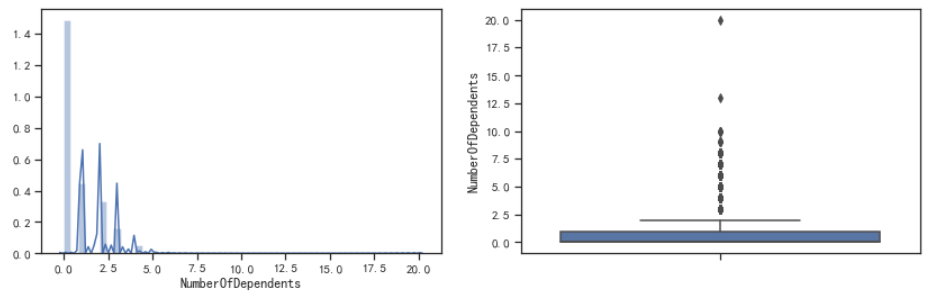• 从箱线图可以很明显地看到存在异常值。那个20特别醒目。。。
cut_num=[-1,0,1,3,5,7,9,15,20]
get_compare_plot(train,feature_plot="NumberOfDependents",cut_num=cut_num,is_qcut=False)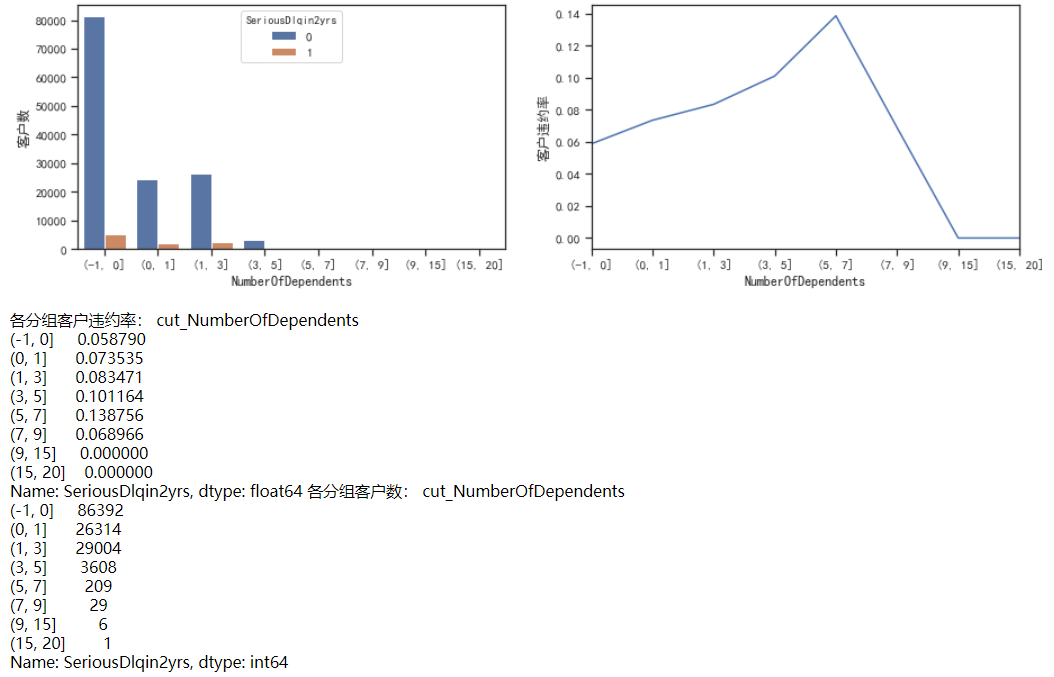• 随着家属人数增加，客户违约率增加。因为家属人数增加，家庭开销增加，需要的开销也就增加。当单身时（家属人数为0）客户违约率最小为0.058。
• 异常值20需删除。
• 将大于5的可分为一箱处理。

3.数据预处理

去除重复值

train.duplicated(keep='first').sum()
609
train.drop_duplicates(keep='first',inplace=True)
train.duplicated(keep='first').sum()
0
train.index = range(train.shape)

异常值处理

def data_deal(data):
#data=data[data['RevolvingUtilizationOfUnsecuredLines']<=2]
data=data[(data['age']>=18)&(data['age']<=100)]
data=data[data['NumberOfTime30-59DaysPastDueNotWorse']<24]
data=data[data['NumberOfTime60-89DaysPastDueNotWorse']<12]
data=data[data['NumberOfTimes90DaysLate']<8]
data=data[data['DebtRatio']<=1]
#data=data[data['NumberOfOpenCreditLinesAndLoans']<50]
data=data[data['NumberRealEstateLoansOrLines']<20]
data=data[data['NumberOfDependents']<20]
return data

train_data = data_deal(train)
test_data = data_deal(test)

填补缺失值

众数填补缺失值

train_data.loc[:,"NumberOfDependents"] = train_data.loc[:,"NumberOfDependents"].fillna(train_data.loc[:,"NumberOfDependents"].median())

随机森林填补缺失值

def fill_missing_rf(X,y,to_fill):

df = X.copy()
fill = df.loc[:,to_fill]
df = pd.concat([df.loc[:,df.columns != to_fill],pd.DataFrame(y)],axis=1)

Ytrain = fill[fill.notnull()]
Ytest = fill[fill.isnull()]

Xtrain = df.loc[Ytrain.index,:]
Xtest = df.loc[Ytest.index,:]

rfr = rfr(n_estimators=200)
rfr = rfr.fit(Xtrain,Ytrain)
Ypredict = rfr.predict(Xtest)

return Ypredict
X = train_data.iloc[:,1:]
y = train_data["SeriousDlqin2yrs"]
y_pred = fill_missing_rf(X,y,"MonthlyIncome")
train_data.loc[train_data.loc[:,"MonthlyIncome"].isnull(),"MonthlyIncome"] = y_pred

数据不平衡处理

X = train_data.iloc[:,1:]
y = train_data.iloc[:,0]

n_sample = X.shape
n_1_sample = y.value_counts()
n_0_sample = y.value_counts()
print('样本个数：{}; 坏样本(1)占{:.2%}; 好样本(0)占{:.2%}'.format(n_sample,n_1_sample/n_sample,n_0_sample/n_sample))

sm = SMOTE(random_state=42)
X,y = sm.fit_sample(X,y)

n_sample_ = X.shape
n_1_sample = pd.Series(y).value_counts()
n_0_sample = pd.Series(y).value_counts()

print('样本个数：{}; 坏样本(1)占{:.2%}; 好样本(0)占{:.2%}'.format(n_sample_,n_1_sample/n_sample_,n_0_sample/n_sample_))

小结

04-13186412-16
08-02
06-142万+
11-11813
07-171795
08-081万+
07-16
01-31
07-232万+
04-268893
11-242237
01-031万+
08-20725
04-191548
04-241万+
03-07357AvenueCyy

¥2 ¥4 ¥6 ¥10 ¥20余额支付 (余额：-- )扫码支付获取中扫码支付点击重新获取扫码支付1.余额是钱包充值的虚拟货币，按照1:1的比例进行支付金额的抵扣。
2.余额无法直接购买下载，可以购买VIP、C币套餐、付费专栏及课程。余额充值